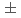To investigate the void coefficient in the Reduced-Moderation Water Reactor (RMWR), an infinite multiplication factor was measured in the FCA-XXII-1 (65V) core. Axial and radial fission rate distributions were measured by micro fission chambers with four different kinds of nuclides. The infinite multiplication factor was derived from the material buckling, which was obtained from both the axial and radial fission rate distributions, and the migration area calculated. The value of it in the test region of the FCA-XXII-1 (65V) core was 1.3440.034. It was compared with the calculation, the ratio of the calculation to the measurement was 1.0080.026. The improvement in measurement accuracy was also considered.